# MCode - 2020.2 English

## Vivado Design Suite Reference Guide: Model-Based DSP Design Using System Generator (UG958)

Document ID
UG958
Release Date
2020-11-18
Version
2020.2 English

This block is listed in the following Xilinx® Blockset libraries: Control Logic, Math, and Index.

The Xilinx MCode block is a container for executing a user-supplied MATLAB function within Simulink. A parameter on the block specifies the M-function name. The block executes the M-code to calculate block outputs during a Simulink simulation. The same code is translated in a straightforward way into equivalent behavioral VHDL/Verilog when hardware is generated.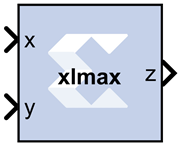The block's Simulink® interface is derived from the MATLAB function signature, and from block mask parameters. There is one input port for each parameter to the function, and one output port for each value the function returns. Port names and ordering correspond to the names and ordering of parameters and return values.

The MCode block supports a limited subset of the MATLAB language that is useful for implementing arithmetic functions, finite state machines, and control logic.

The MCode block has the following three primary coding guidelines that must be followed:

• All block inputs and outputs must be of Xilinx fixed-point type.
• The block must have at least one output port.
• The code for the block must exist on the MATLAB path or in the same directory as the directory as the model that uses the block.

The example described below consists of a function `xlmax` which returns the maximum of its inputs. The second illustrates how to do simple arithmetic. The third shows how to build a finite state machine.

## Configuring an MCode Block

The MATLAB Function parameter of an MCode block specifies the name of the block's M- code function. This function must exist in one of the three locations at the time this parameter is set. The three possible locations are:

• The directory where the model file is located.
• A subdirectory of the model directory named private.
• A directory in the MATLAB path.

The block icon displays the name of the M-function. To illustrate these ideas, consider the file `xlmax.m` containing function `xlmax`:

``````
function z = xlmax(x, y)
if x > y
z = x;
else
z = y;
end
``````

An MCode block based on the function `xlmax` will have input ports `x` and `y` and output port `z`.

The following figure shows how to set up an MCode block to use function `xlmax`.

Figure 1. xlmax function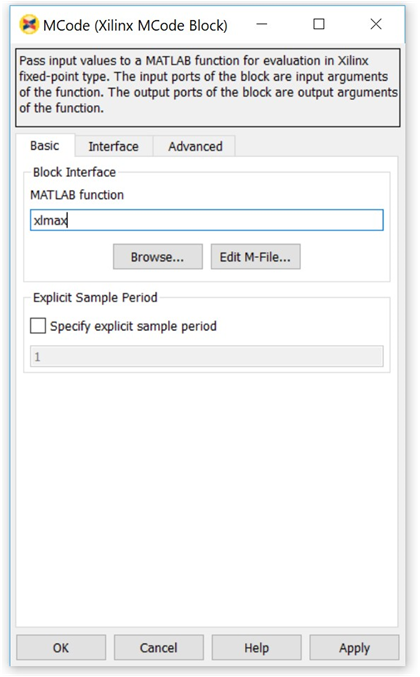Once the model is compiled, the xlmax MCode block will appear like the block illustrated below.

Figure 2. xlmax MCode block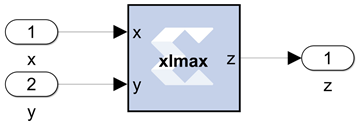## MATLAB Language Support

The MCode block supports the following MATLAB language constructs:

• Assignment statements
• Simple and compound `if/else/elseif` end statements
• `switch` statements
• Arithmetic expressions involving only addition and subtraction
• Subtraction
• Multiplication
• Division by a power of two
• Relational operators:
 < Less than <= Less than or equal to > Greater than >= Greater than or equal to == Equal to ~= Not equal to
• Logical operators:
 `& ` And `| ` Or `~ ` Not

The MCode block supports the following MATLAB functions.

• Type conversion. The only supported data type is `xfix`, the Xilinx fixed-point type. The `xfix()` type conversion function is used to convert to this type. The conversion is done implicitly for integers but must be done explicitly for floating point constants. All values must be scalar; arrays are not supported.
• Functions that return `xfix` properties:
 `xl_nbits() ` Returns number of bits `xl_binpt() ` Returns binary point position `xl_arith() ` Returns arithmetic type
• Bit-wise logical functions:
 `xl_and() ` Bit-wise and `xl_or() ` Bit-wise or `xl_xor() ` Bit-wise xor `xl_not() ` Bit-wise not
• Shift functions: `xl_lsh()` and `xl_rsh() `
• Slice function: `xl_slice() `
• Concatenate function: `xl_concat() `
• Reinterpret function: `xl_force() `
• Internal state variables: `xl_state() `
• MATLAB Functions:
 `disp() ` Displays variable values `error() ` Displays message and abort function `isnan() ` Tests whether a number is NaN `NaN() ` Returns Not-a-Number `num2str() ` Converts a number to string `ones(1,N) ` Returns 1-by-N vector of ones `pi() ` Returns pi `zeros(1,N) ` Returns 1-by-N vector of zeros
Data Types

There are three kinds of `xfix` data types: unsigned fixed-point (`xlUnsigned`), signed fixed-point(`xlSigned`), and boolean (`xlBoolean`). Arithmetic operations on these data types produce signed and unsigned fixed-point values. Relational operators produce a boolean result. Relational operands can be any `xfix` type, provided the mixture of types makes sense. Boolean variables can be compared to boolean variables, but not to fixed-point numbers; boolean variables are incompatible with arithmetic operators. Logical operators can only be applied to boolean variables. Every operation is performed in full precision, for example, with the minimum precision needed to guarantee that no information is lost.

### Literal Constants

Integer, floating-point, and boolean literals are supported. Integer literals are automatically converted to `xfix` values of appropriate width having a binary point position at zero. Floating-point literals must be converted to the `xfix` type explicitly with the `xfix()` conversion function. The predefined MATLAB values `true` and `false` are automatically converted to boolean literals.

### Assignment

The left-hand side of an assignment can only contain one variable. A variable can be assigned more than once.

### Control Flow

The conditional expression of an `if` statement must evaluate to a boolean. Switch statements can contain a `case` clause and an `otherwise` clause. The types of a switch selector and its cases must be compatible; thus, the selector can be boolean provided its cases are. All cases in a `switch` must be constant; equivalently, no `case` can depend on an input value.

When the same variable is assigned in several branches of a control statement, the types being assigned must be compatible. For example,

``````
if (u > v)
x = a;
else
x = b;
end
``````

is acceptable only if `a` and `b` are both boolean or both arithmetic.

Constant Expressions

An expression is constant provided its value does not depend on the value of any input argument. Thus, for example, the variable `c` defined by

``````
a = 1;
b = a + 2;
c = xfix({xlSigned, 10, 2}, b + 3.345);
``````

can be used in any context that demands a constant.

### xfix() Conversion

The `xfix()` conversion function converts a `double` to an `xfix`, or changes one `xfix` into another having different characteristics. A call on the conversion function looks like the following

``````
x = xfix(type_spec, value)
``````

Here `x` is the variable that receives the `xfix`. type_spec is a cell array that specifies the type of `xfix` to create, and value is the value being operated on. The` value` can be floating point or `xfix` type. The type_spec cell array is defined using curly braces in the usual MATLAB method. For example,

``````
xfix({xlSigned, 20, 16, xlRound, xlWrap}, 3.1415926)
``````

returns an `xfix` approximation to `pi`. The approximation is signed, occupies 20 bits (16 fractional), quantizes by rounding, and wraps on overflow.

The type_spec consists of 1, 3, or 5 elements. Some elements can be omitted. When elements are omitted, default element settings are used. The elements specify the following properties (in the order presented): `data type`, `width`, ```binary point position```, `quantization mode`, and `overflow mode`. The `data type` can be `xlBoolean`, `xlUnsigned`, or `xlSigned`. When the type is `xlBoolean`, additional elements are not needed (and must not be supplied). For other types, `width` and `binary point position` must be supplied. The `quantization` and `overflow mode`s are optional, but when one is specified, the other must be as well. Three values are possible for quantization: `xlTruncate`, `xlRound`, and `xlRoundBanker`. The default is `xlTruncate`. Similarly, three values are possible for overflow: `xlWrap`, `xlSaturate`, and `xlThrowOverflow`. For `xlThrowOverflow`, if an overflow occurs during simulation, an exception occurs.

All values in a type_spec must be known at compilation time; equivalently, no type_spec value can depend on an input to the function.

The following is a more elaborate example of an `xfix()` conversion:

``````
width = 10, binpt = 4;
z = xfix({xlUnsigned, width, binpt}, x + y);
``````

This assignment to `x` is the result of converting `x + y` to an unsigned fixed-point number that is 10 bits wide with 4 fractional bits using `xlTruncate` for quantization and `xlWrap` for overflow.

If several `xfix()` calls need the same type_spec value, you can assign the type_spec to a variable, then use the variable for `xfix()` calls. For example, the following is allowed:

``````
proto = {xlSigned, 10, 4};
x = xfix(proto, a);
y = xfix(proto, b);
``````
xfix Properties: xl_arith, xl_nbits, and xl_binpt

Each `xfix` number has three properties: the arithmetic type, the bit width, and the binary point position. The MCode blocks provide three functions to get these properties of a fixed- point number. The results of these functions are constants and are evaluated when Simulink compiles the model.

Function `a = xl_arith(x)` returns the arithmetic type of the input number `x`. The return value is either `1`, `2`, or `3` for `xlUnsigned`, `xlSigned`, or `xlBoolean` respectively.

Function `n = xl_nbits(x)` returns the width of the input number `x`.

Function `b = xl_binpt(x)` returns the binary point position of the input number `x`.

Bit-wise Operators: xl_or, xl_and, xl_xor, and xl_not

The MCode block provides four built-in functions for bit-wise logical operations: `xl_or`, `xl_and`, `xl_xor`, and `xl_not`.

Function `xl_or`, `xl_and`, and `xl_xor` perform bit-wise logical or, and, and xor operations respectively. Each function is in the form of

``````
x = xl_op(a, b, …).
``````

Each function takes at least two fixed-point numbers and returns a fixed-point number. All the input arguments are aligned at the binary point position.

Function `xl_not` performs a bit-wise logical not operation. It is in the form of ```x = xl_not(a)```. It only takes one `xfix` number as its input argument and returns a fixed- point number.

The following are some examples of these function calls:

``````
X = xl_and(a, b);
Y = xl_or(a, b, c);
Z = xl_xor(a, b, c, d);
N = xl_not(x);
``````
Shift Operators: xl_rsh, and xl_lsh

Functions `xl_lsh` and `xl_rsh` allow you to shift a sequence of bits of a fixed-point number. The function is in the form:

`x = xl_lsh(a, n)` and `x = xl_rsh(a, n)` where `a` is a `xfix` value and `n` is the number of bits to shift.

Left or right shift the fixed-point number by `n` number of bits. The right shift (`xl_rsh`) moves the fixed-point number toward the least significant bit. The left shift (`xl_lsh`) function moves the fixed-point number toward the most significant bit. Both shift functions are a full precision shift. No bits are discarded and the precision of the output is adjusted as needed to accommodate the shifted position of the binary point.

Here are some examples:

``````
% left shift a 5 bits
a = xfix({xlSigned, 20, 16, xlRound, xlWrap}, 3.1415926)
b = xl_rsh(a, 5);
``````

The output `b` is of type `xlSigned` with 21 bits and the binary point located at bit 21.

Slice Function: xl_slice

Function `xl_slice` allows you to access a sequence of bits of a fixed-point number. The function is in the form:

``````
x = xl_slice(a, from_bit, to_bit).
``````

Each bit of a fixed-point number is consecutively indexed from zero for the LSB up to the MSB. For example, given an 8-bit wide number with binary point position at zero, the LSB is indexed as 0 and the MSB is indexed as 7. The block will throw an error if the `from_bit` or `to_bit` arguments are out of the bit index range of the input number. The result of the function call is an unsigned fixed-point number with zero binary point position.

Here are some examples:

``````
% slice 7 bits from bit 10 to bit 4
b = xl_slice(a, 10, 4);
% to get MSB
c = xl_slice(a, xl_nbits(a)-1, xl_nbits(a)-1);
``````
Concatenate Function: xl_concat

Function ```x = xl_concat(hi, mid, ..., low)``` concatenates two or more fixed-point numbers to form a single fixed-point number. The first input argument occupies the most significant bits, and the last input argument occupies the least significant bits. The output is an unsigned fixed-point number with binary point position at zero.

Reinterpret Function: xl_force

Function ```x = xl_force(a, arith, binpt)``` forces the output to a new type with `arith` as its new arithmetic type and `binpt` as its new binary point position. The `arith` argument can be one of `xlUnsigned`, `xlSigned`, or `xlBoolean`. The `binpt` argument must be from 0 to the bit width inclusively. Otherwise, the block will throw an error.

State Variables: xl_state

An MCode block can have internal state variables that hold their values from one simulation step to the next. A state variable is declared with the MATLAB keyword persistent and must be initially assigned with an `xl_state` function call.

The following code models a 4-bit accumulator:

``````
function q = accum(din, rst)
init = 0;

persistent s, s = xl_state(init, {xlSigned, 4, 0});
q = s;
if rst
s = init;
else
s = s + din;
end
``````

The state variable `s` is declared as persistent, and the first assignment to `s` is the result of the `xl_state` invocation. The `xl_state` function takes two arguments. The first is the initial value and must be a constant. The second is the precision of the state variable. It can be a type cell array as described in the `xfix` function call. It can also be an `xfix` number. In the above code, if ```s = xl_state(init, din)```, then state variable s will use `din` as the precision. The `xl_state` function must be assigned to a persistent variable.

The `xl_state` function behaves in the following way:

1. In the first cycle of simulation, the `xl_state` function initializes the state variable with the specified precision.
2. In the following cycles of simulation, the `xl_state `function retrieves the state value left from the last clock cycle and assigns the value to the corresponding variable with the specified precision.

`v = xl_state(init, precision)` returns the value of a state variable. The first input argument `init` is the initial value, the second argument `precision` is the precision for this state variable. The argument `precision` can be a cell arrary in the form of ```{type, nbits, binpt}``` or `{type, nbits, binpt, quantization,overflow}`. The `precision` argument can also be an `xfix` number.

`v = xl_state(init, precision, maxlen)` returns a vector object. The vector is initialized with `init` and will have `maxlen` for the maximum length it can be. The vector is initialized with `init`. For example, `v = xl_state(zeros(1, 8), prec, 8)` creates a vector of 8 zeros, `v = xl_state([], prec, 8)` creates an empty vector with 8 as maximum length, `v = xl_state(0, prec, 8)` creates a vector of one zero as content and with 8 as the maximum length.

Conceptually, a vector state variable is a double ended queue. It has two ends, the front which is the element at address 0 and the back which is the element at length – 1.

Methods available for vector are:

 `val = v(idx); ` Returns the value of element at address idx. `v(idx) = val; ` Assigns the element at address idx with val. `f = v.front; ` Returns the value of the front end. An error is thrown if the vector is empty. `v.push_front(val); ` Pushes val to the front and then increases the vector length by 1. An error is thrown if the vector is full. `v.pop_front; ` Pops one element from the front and decreases the vector length by 1. An error is thrown if the vector is empty. `b = v.back; ` Returns the value of the back end. An error is thrown if the vector is empty. `v.push_back(val); ` Pushes val to the back and the increases the vector length by 1. An error is thrown if the vector is full. `v.pop_back; ` Pops one element from the back and decreases the vector length by 1. An error is thrown if the vector is empty. `v.push_front_pop_back(val); ` Pushes val to the front and pops one element out from the back. It's a shift operation. The length of the vector is unchanged. The vector cannot be empty to perform this operation. `full = v.full; ` Returns `true` if the vector is full, otherwise, `false`. `empty = v.empty; ` Returns `true` if the vector is empty, otherwise, `false`. `len = v.length; ` Returns the number of elements in the vector.

A method of a vector that queries a state variable is called a query method. It has a return value. The following methods are query method: `v(idx)`, `v.front`, `v.back`, `v.full`, `v.empty`, `v.length`, `v.maxlen`. A method of a vector that changes a state variable is called an update method. An update method does not return any value. The following methods are update methods: `v(idx) = val`, `v.push_front(val)`, `v.pop_front`, `v.push_back(val)`, `v.pop_back`, and `v.push_front_pop_back(val)`. All query methods of a vector must be invoked before any update method is invocation during any simulation cycle. An error is thrown during model compilation if this rule is broken.

The MCode block can map a vector state variable into a vector of registers, a delay line, an addressable shift register, a single port ROM, or a single port RAM based on the usage of the state variable. The `xl_state` function can also be used to convert a MATLAB 1-D array into a zero-indexed constant array. If the MCode block cannot map a vector state variable into an FPGA, an error message is issued during model netlist time. The following are examples of using vector state variables.

Delay Line

The state variable in the following function is mapped into a delay line.

``````
function q = delay(d, lat)
persistent r, r = xl_state(zeros(1, lat), d, lat);
q = r.back;
r.push_front_pop_back(d);``````

Line of Registers

The state variable in the following function is mapped into a line of registers.

``````
function s = sum4(d)
persistent r, r = xl_state(zeros(1, 4), d);
S = r(0) + r(1) + r(2) + r(3);
r.push_front_pop_back(d);``````

Vector of Constants

The state variable in the following function is mapped into a vector of constants.

``````
function s = myadd(a, b, c, d, nbits, binpt)
p = {xlSigned, nbits, binpt, xlRound, xlSaturate};
persistent coef, coef = xl_state([3, 7, 3.5, 6.7], p);
s = a*coef(0) + b*coef(1) + c*coef(2) + c*coef(3);``````

The state variable in the following function is mapped into an addressable shift register.

``````
persistent r, r = xl_state(zeros(1, depth), d);
if en
r.push_front_pop_back(d);
end

``````

## Single Port ROM

The state variable in the following function is mapped into a single port ROM.

``````
proto = {arith, nbits, binpt};
persistent mem, mem = xl_state(contents, proto);
``````
Single Port RAM

The state variable in the following function is mapped to a single port RAM in fabric (Distributed RAM).

``````
function dout = ram(addr, we, din, depth, nbits, binpt)
proto = {xlSigned, nbits, binpt};
persistent mem, mem = xl_state(zeros(1, depth), proto);
if we
end
``````

The state variable in the following function is mapped to BlockRAM as a single port RAM.

``````
function dout = ram(addr, we, din, depth, nbits, binpt,ram_enable)
proto = {xlSigned, nbits, binpt};
persistent mem, mem = xl_state(zeros(1, depth), proto);
persistent dout_temp, dout_temp = xl_state(0,proto);
dout = dout_temp;
if we
end

``````

## MATLAB Functions

disp()

Displays the expression value. In order to see the printing on the MATLAB console, the option Enable printing with disp must be checked on the Advanced tab of the MCode block parameters dialog box. The argument can be a string, an `xfix` number, or an MCode state variable. If the argument is an `xfix` number, it will print the type, binary value, and double precision value. For example, if variable `x` is assigned with `xfix({xlSigned, 10, 7}, 2.75)`, the `disp(x)` will print the following line:

``````
type: Fix_10_7, binary: 010.1100000, double: 2.75
``````

If the argument is a vector state variable, `disp()` will print out the type, maximum length, current length, and the binary and double values of all the elements. For each simulation step, when Enable printing with disp is on and when a disp() function is invoked, a title line is printed for the corresponding block. The title line includes the block name, Simulink simulation time, and FPGA clock number.

The following MCode function shows several examples of using the `disp()` function.

``````
function x = testdisp(a, b)
persistent dly, dly = xl_state(zeros(1, 8), a);
persistent rom, rom = xl_state([3, 2, 1, 0], a);
disp('Hello World!');
disp(['num2str(dly) is ', num2str(dly)]);
disp('disp(dly) is ');
disp(dly);
disp('disp(rom) is ');
disp(rom);
a2 = dly.back;
dly.push_front_pop_back(a);
x = a + b;
disp(['a = ', num2str(a), ', ', ...
'b = ', num2str(b), ', ', ...
'x = ', num2str(x)]);
disp(num2str(true));
disp('disp(10) is');
disp(10);
disp('disp(-10) is');
disp(-10);
disp('disp(a) is ');
disp(a);
disp('disp(a == b)');
disp(a==b);
``````

The following lines are the result for the first simulation step.

``````
xlmcode_testdisp/MCode (Simulink time: 0.000000, FPGA clock: 0)
Hello World!
num2str(dly) is [0.000000, 0.000000, 0.000000, 0.000000, 0.000000, 0.000000,
0.000000, 0.000000]
disp(dly) is
type: Fix_11_7,
maxlen: 8,
length: 8,
0: binary 0000.0000000, double 0.000000,
1: binary 0000.0000000, double 0.000000,
2: binary 0000.0000000, double 0.000000,
3: binary 0000.0000000, double 0.000000,
4: binary 0000.0000000, double 0.000000,
5: binary 0000.0000000, double 0.000000,
6: binary 0000.0000000, double 0.000000,
7: binary 0000.0000000, double 0.000000,
disp(rom) is
type: Fix_11_7,
maxlen: 4,
length: 4,
0: binary 0011.0000000, double 3.0,
1: binary 0010.0000000, double 2.0,
2: binary 0001.0000000, double 1.0,
3: binary 0000.0000000, double 0.0,
a = 0.000000, b = 0.000000, x = 0.000000
1
disp(10) is
type: UFix_4_0, binary: 1010, double: 10.0
disp(-10) is
type: Fix_5_0, binary: 10110, double: -10.0
disp(a) is
type: Fix_11_7, binary: 0000.0000000, double: 0.000000
disp(a == b)
type: Bool, binary: 1, double: 1``````
error()

Displays message and abort function. See MATLAB help on this function for more detailed information. Message formatting is not supported by the MCode block. For example:

``````if latency <=0
error('latency must be a positive');
end
``````
isnan()

Returns true for Not-a-Number. `isnan(X)` returns true when `X` is Not-a-Number. `X` must be a scalar value of double or Xilinx fixed-point number. This function is not supported for vectors or matrices. For example:

``````
if isnan(incr) & incr == 1
cnt = cnt + 1;
end
``````
NaN()

The `NaN()` function generates an IEEE arithmetic representation for Not-a-Number. A NaN is obtained as a result of mathematically undefined operations like 0.0/0.0 and inf-inf. NaN(1,N) generates a 1-by-N vector of NaN values. Here are examples of using NaN.

``````if x < 0
z = NaN;
else
z = x + y;
end
``````
num2Str()

Converts a number to a string. `num2str(X)` converts the `X` into a string. `X` can be a scalar value of double, a Xilinx fixed-point number, or a vector state variable. The default number of digits is based on the magnitude of the elements of `X`. Here's an example of `num2str`:

``````
if opcode <=0 | opcode >= 10
error(['opcode is out of range: ', num2str(opcode)]);
end``````
ones()

The `ones()` function generates a specified number of one values. `ones(1,N)` generates a 1-by-N vector of ones. `ones(M,N)` where `M` must be 1. It's usually used with `xl_state()` function call. For example, the following line creates a 1-by-4 vector state variable initialized to [1, 1, 1, 1].

``````
persitent m, m = xl_state(ones(1, 4), proto)
``````
zeros()

The `zeros()` function generates a specified number of zero values. `zeros(1,N)` generates a 1-by-N vector of zeros. `zero(M,N)` where `M` must be 1. It's usually used with `xl_state()` function call. For example, the following line creates a 1-by-4 vector state variable initialized to [0, 0, 0, 0].

``````
persitent m, m = xl_state(zeros(1, 4), proto)
``````
FOR Loop

`FOR` statement is fully unrolled. The following function sums `n` samples.

``````
function q = sum(din, n)
persistent regs, regs = xl_state(zeros(1, 4), din);
q = reg(0);
for i = 1:n-1
q = q + reg(i);
end
regs.push_front_pop_back(din);
``````

The following function does a bit reverse.

``````
function q = bitreverse(d)
q = xl_slice(d, 0, 0);
for i = 1:xl_nbits(d)-1
q = xl_concat(q, xl_slice(d, i, i));
end
``````
Variable Availability

MATLAB code is sequential (for example, statements are executed in order). The MCode block requires that every possible execution path assigns a value to a variable before it is used (except as a left-hand side of an assignment). When this is the case, we say the variable is available for use. The MCode block will throw an error if its M-code function accesses unavailable variables.

Consider the following M-code:

``````
function [x, y, z] = test1(a, b)
x = a;
if a>b
x = a + b; y = a;
end
switch a
case 0
z = a + b;
case 1
z = a - b;
end
``````

Here `a`, `b`, and `x` are available, but `y` and `z` are not. Variable `y` is not available because the if statement has no else, and variable `z` is not available because the switch statement has no otherwise part.

### DEBUG MCode

There are two ways to debug your MCode. One is to insert `disp()` functions in your code and enable printing; the other is to use the MATLAB debugger. For usage of the disp() function, see the disp() section in this topic.

If you want to use the MATLAB debugger, you need to check the Enable MATLAB debugging option on the Advanced tab of the MCode block parameters dialog box. Then you can open your MATLAB function with the MATLAB editor, set break points, and debug your M-function. Just be aware that every time you modify your script, you need to execute a `clear functions` command in the MATLAB console.

To start debugging your M-function, you need to first check the Enable MATLAB debugging check box on the Advanced tab of the MCode block parameters dialog, then click the OK or Apply button.

Figure 8. Enable MATLAB Debugging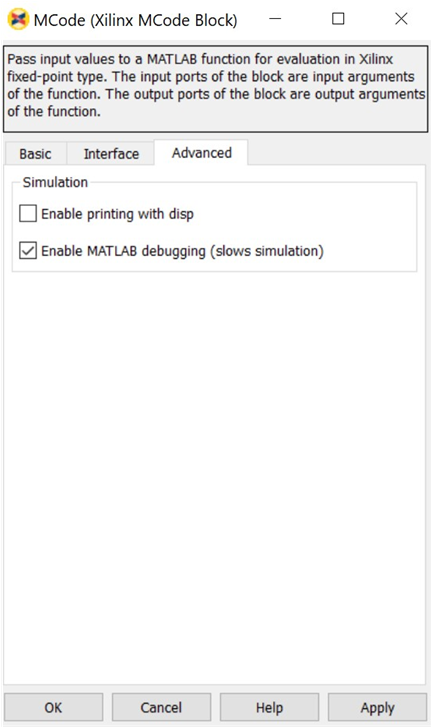Now you can edit the M-file with the MATLAB editor and set break points as needed.

Figure 9. Set Break Points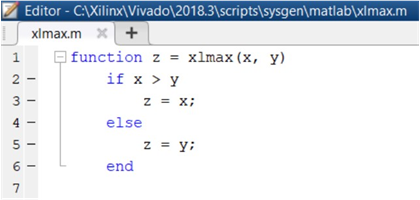During the Simulink simulation, the MATLAB debugger will stop at the break points you set when the break points are reached.

Figure 10. Stopping at Break Point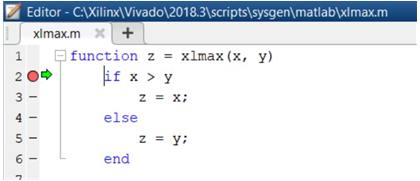When debugging, you can also examine the values of the variables by typing the variable names in the MATLAB console.

Figure 11. Examining Variable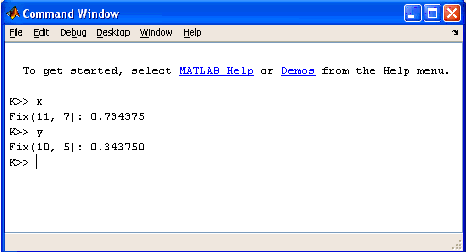There is one special case to consider when the function for an MCode block is executed from the MATLAB debugger. A `switch/case` expression inside an MCode block must be type `xfix`, however, executing a `switch/case` expression from the MATLAB console requires that the expression be a `double` or `char`. To facilitate execution in the MATLAB console, a call to `double()` must be added. For example, consider the following:

``````
switch i
case 0
x = 1
case 1
x = 2
end
``````

where `i` is type `xfix`. To run from the console this code must changed to

``````
switch double(i)
case 0
x = 1
case 1
x = 2
end
``````

The `double()` function call only has an effect when the M code is run from the console. The MCode block ignores the `double()` call.

Passing Parameters

It is possible to use the same M-function in different MCode blocks, passing different parameters to the M-function so that each block can behave differently. This is achieved by binding input arguments to some values. To bind the input arguments, select the Interface tab on the block GUI. After you bind those arguments to some values, these M-function arguments will not be shown as input ports of the MCode block.

Consider for example, the following M-function:

``````
function dout = xl_sconvert(din, nbits, binpt)
proto = {xlSigned, nbits, binpt};
dout = xfix(proto, din);
``````

The following figures shows how the bindings are set for the din input of two separate `xl_sconvert` blocks.

Figure 12. din Bindings, Example 1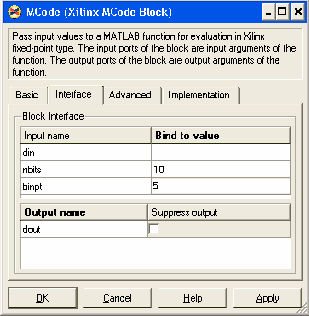Figure 13. din Bindings, Example 2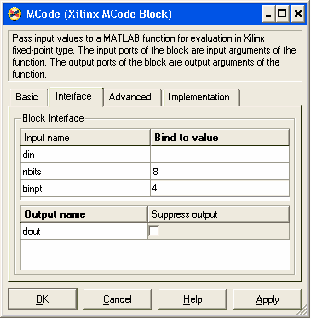The following figure shows the block diagram after the model is compiled.

Figure 14. Block Diagram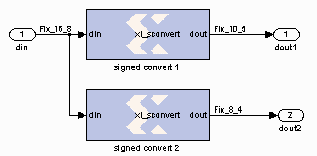The parameters can only be of type double or they can be logical numbers.

Optional Input Ports

The parameter passing mechanism allows the MCode block to have optional input ports. Consider for example, the following M-function:

``````
function s = xl_m_addsub(a, b, sub)
if sub
s = a - b;
else
s = a + b;
end
``````

If `sub` is set to be `false`, the MCode block that uses this M-function will have two input ports `a` and `b` and will perform full precision addition. If it is set to an empty cell array {}, the block will have three input ports `a`, `b`, and `sub` and will perform full precision addition or subtraction based on the value of input port `sub`.

The following figure shows the block diagram of two blocks using the same `xl_m_addsub` function, one having two input ports and one having three input ports.

Figure 15. Two Blocks Using Same xl_m_addsub Function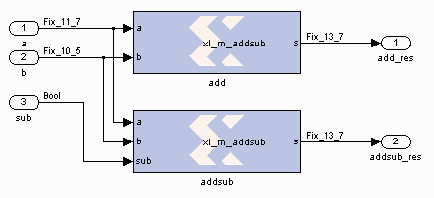Constructing a State Machine

There are two ways to build a state machine using an MCode block. One way is to specify a stateless transition function using a MATLAB function and pair an MCode block with one or more state register blocks. Usually the MCode block drives a register with the value representing the next state, and the register feeds back the current state into the MCode block. For this to work, the precision of the state output from the MCode block must be static, that is, independent of any inputs to the block. Occasionally you might find you need to use `xfix()` conversions to force static precision. The following code illustrates this:

``````
function nextstate = fsm1(currentstate, din)
% some other code
nextstate = currentstate;
switch currentstate
case 0, if din==1, nextstate = 1; end
end
% a xfix call should be used at the end
nextstate = xfix({xlUnsigned, 2, 0}, nextstate);
``````

Another way is to use state variables. The above function can be re-written as follows:

``````
function currentstate = fsm1(din)
persistent state, state=xl_state(0,{xlUnsigned,2,0});
currentstate = state;
switch double(state)
case 0, if din==1; state = 1; end
end
``````
Reset and Enable Signals for State Variables

The MCode block can automatically infer register reset and enable signals for state variables when conditional assignments to the variables contain two or fewer branches.

For example, the following M-code infers an enable signal for conditional assignment of persistent state variable `r1`:

``````
function myFn = aFn(en, a)
persistent r1, r1 = xl_state(0, {xlUnsigned, 2, 0});
myFn = r1;
if en
r1 = r1 + a
else
r1 = r1
end
``````

There are two branches in the conditional assignment to persistent state variable `r1`. A register is used to perform the conditional assignment. The input of the register is connected to `r1 + a`, the output of the register is `r1`. The register's enable signal is inferred; the enable signal is connected to `en`, when `en` is asserted. Persistent state variable `r1` is assigned to `r1 + a` when `en` evaluates to `false`, the enable signal on the register is de-asserted resulting in the assignment of `r1` to `r1`.

The following M-code will also infer an enable signal on the register used to perform the conditional assignment:

``````
function myFn = aFn(en, a)
persistent r1, r1 = xl_state(0, {xlUnsigned, 2, 0});
myFn = r1;
if en
r1 = r1 + a
end
``````

An enable is inferred instead of a reset because the conditional assignment of persistent state variable `r1` is to a non-constant value, ```r1 + a```.

If there were three branches in the conditional assignment of persistent state variable `r1`, the enable signal would not be inferred. The following M-code illustrates the case where there are three branches in the conditional assignment of persistent state variable `r1` and the enable signal is not inferred:

``````
function myFn = aFn(en, en2, a, b)
persistent r1, r1 = xl_state(0, {xlUnsigned, 2, 0});
if en
r1 = r1 + a
elseif en2
r1 = r1 + b
else
r1 = r1
end``````

The reset signal can be inferred if a persistent state variable is conditionally assigned to a constant; the reset is synchronous. Consider the following M-code example which infers a reset signal for the assignment of persistent state variable `r1` to `init`, a constant, when `rst` evaluates to true and `r1 + 1` otherwise:

``````
function myFn = aFn(rst)
persistent r1, r1 = xl_state(0, {xlUnsigned, 4, 0});
myFn = r1;
init = 7;
if (rst)
r1 = init
else
r1 = r1 + 1
end
``````

The M-code example above which infers reset can also be written as:

``````
function myFn = aFn(rst)
persistent r1, r1 = xl_state(0, {xlUnsigned,4,0});
init = 1;
myFn = r1;
r1 = r1 +1
if (rst)
r1 = init
end
``````

In both code examples above, the reset signal of the register containing persistent state variable `r1` is assigned to `rst`. When `rst` evaluates to `true`, the register's reset input is asserted and the persistent state variable is assigned to constant `init`. When `rst` evaluates to `false`, the register's reset input is de-asserted and persistent state variable `r1` is assigned to `r1 + 1`. Again, if the conditional assignment of a persistent state variable contains three or more branches, a reset signal is not inferred on the persistent state variable's register.

It is possible to infer reset and enable signals on the register of a single persistent state variable. The following M-code example illustrates simultaneous inference of reset and enable signals for the persistent state variable `r1`:

``````
function myFn = aFn(rst,en)
persistent r1, r1 = xl_state(0, {xlUnsigned, 4, 0});
myFn = r1;
init = 0;
if rst
r1 = init
else
if en
r1 = r1 + 1
end
end
``````

The reset input for the register of persistent state variable `r1` is connected to `rst`; when `rst` evaluates to `true`, the register's reset input is asserted and `r1` is assigned to `init`. The enable input of the register is connected to `en`; when `en` evaluates to `true`, the register's enable input is asserted and `r1` is assigned to ```r1 + 1```. It is important to note that an inferred reset signal takes precedence over an inferred enable signal regardless of the order of the conditional assignment statements. Consider the second code example above; if both `rst` and `en` evaluate to `true`, persistent state variable `r1` would be assigned to `init`.

Inference of reset and enable signals also works for conditional assignment of persistent state variables using switch statements, provided the switch statements contain two or less branches.

The MCode block performs dead code elimination and constant propagation compiler optimizations when generating code for the FPGA. This can result in the inference of reset and/or enable signals in conditional assignment of persistent state variables, when one of the branches is never executed. For this to occur, the conditional must contain two branches that are executed after dead code is eliminated, and constant propagation is performed.

Inferring Registers

Registers are inferred in hardware by using persistent variables, however, the right coding style must be used. Consider the two code segments in the following function:

``````
function [out1, out2] = persistent_test02(in1, in2)
persistent ff1, ff1 = xl_state(0, {xlUnsigned, 2, 0});
persistent ff2, ff2 = xl_state(0, {xlUnsigned, 2, 0});
%code segment 1
out1 = ff1; %these two statements infer a register for ff1
ff1  = in1;
%code segment 2
ff2  = in2; %these two statements do NOT infer a register for ff2
out2 = ff2;
end
``````

In code segment 1, the value of persistent variable ff1 is assigned to out1. Since ff1 is persistent , it is assumed that its current value was assigned in the previous cycle. In the next statement, the value of in1 is assigned to ff1 so it can be saved for the next cycle. This infers a register for ff1.

In code segment 2, the value of in2 is first assigned to persistent variable ff2, then assigned to out2. These two statements can be completed in one cycle, so a register is not inferred. If you need to insert delay into combinational logic, refer to the next topic.

Pipelining Combinational Logic

The generated FPGA bitstream for an MCode block might contain many levels of combinational logic and hence a large critical path delay. To allow a downstream logic synthesis tool to automatically pipeline the combinational logic, you can add delay blocks before the MCode block inputs or after the MCode block outputs. These delay blocks should have the parameter Implement using behavioral HDL set, which instructs the code generator to implement delay with synthesizable HDL. You can then instruct the downstream logic synthesis tool to implement register re-timing or register balancing. As an alternative approach, you can use the vector state variables to model delays.

Shift Operations with Multiplication and Division

The MCode block can detect when a number is multiplied or divided by constants that are powers of two. If detected, the MCode block will perform a shift operation. For example, multiplying by 4 is equivalent to left shifting 2 bits and dividing by 8 is equivalent to right shifting 3 bits. A shift is implemented by adjusting the binary point, expanding the `xfix` container as needed. For example, a `Fix_8_4` number multiplied by 4 will result in a `Fix_8_2` number, and a `Fix_8_4` number multiplied by 64 will result in a `Fix_10_0` number.

Using the xl_state Function with Rounding Mode

The `xl_state` function call creates an `xfix` container for the state variable. The container's precision is specified by the second argument passed to the `xl_state` function call. If precision uses `xlRound` for its rounding mode, hardware resources is added to accomplish the rounding. If rounding the initial value is all that is required, an `xfix` call to round a constant does not require additional hardware resources. The rounded value can then be passed to the `xl_state` function. For example:

``````
init = xfix({xlSigned,8,5,xlRound,xlWrap}, 3.14159);
persistent s, s = xl_state(init, {xlSigned, 8, 5});
``````

## Block Parameters

The block parameters dialog box can be invoked by double-clicking the block icon in a Simulink® model.

Figure 16. Block Parameters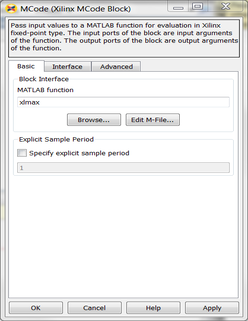As described earlier in this topic, the MATLAB function parameter on an MCode block tells the name of the block's function, and the Interface tab specifies a list of constant inputs and their values.

Other parameters used by this block are explained in the topic Common Options in Block Parameter Dialog Boxes.